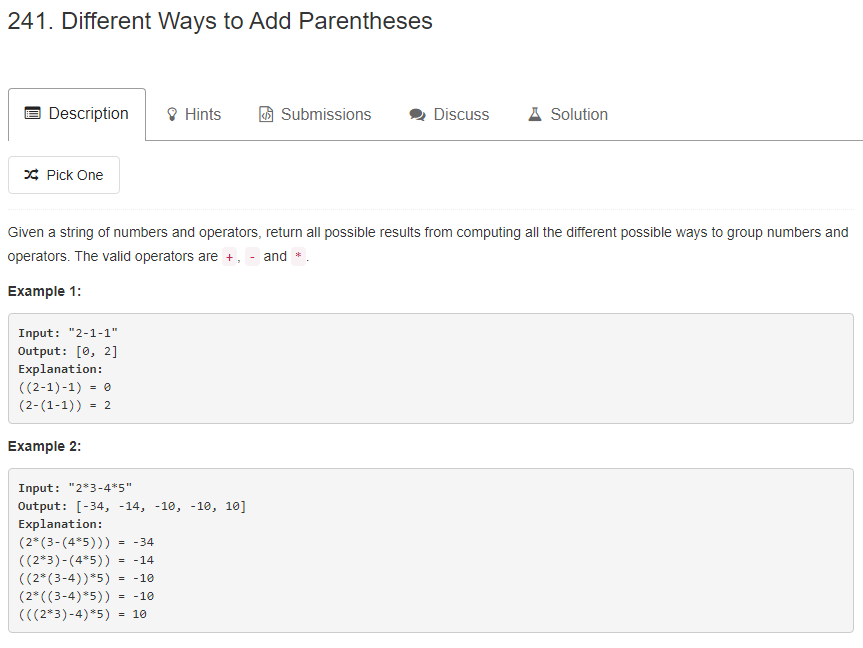# 题目描述（中等难度）# 解法一 递归

2 * 3 - 4 * 5 为例。

23 - 4 * 5 两部分，中间是 * 号相连。

2 * 34 * 5 两部分，中间是 - 号相连。

2 * 3 - 45 两部分，中间是 * 号相连。

2 的解就是 3 - 4 * 5 的解就是 [-5, -17]

public List<Integer> diffWaysToCompute(String input) {
if (input.length() == 0) {
return new ArrayList<>();
}
List<Integer> result = new ArrayList<>();
int num = 0;
//考虑是全数字的情况
int index = 0;
while (index < input.length() && !isOperation(input.charAt(index))) {
num = num * 10 + input.charAt(index) - '0';
index++;
}
//将全数字的情况直接返回
if (index == input.length()) {
return result;
}

for (int i = 0; i < input.length(); i++) {
//通过运算符将字符串分成两部分
if (isOperation(input.charAt(i))) {
List<Integer> result1 = diffWaysToCompute(input.substring(0, i));
List<Integer> result2 = diffWaysToCompute(input.substring(i + 1));
//将两个结果依次运算
for (int j = 0; j < result1.size(); j++) {
for (int k = 0; k < result2.size(); k++) {
char op = input.charAt(i);
}
}
}
}
return result;
}

private int caculate(int num1, char c, int num2) {
switch (c) {
case '+':
return num1 + num2;
case '-':
return num1 - num2;
case '*':
return num1 * num2;
}
return -1;
}

private boolean isOperation(char c) {
return c == '+' || c == '-' || c == '*';
}


//添加一个 map
HashMap<String,List<Integer>> map = new HashMap<>();
public List<Integer> diffWaysToCompute(String input) {
if (input.length() == 0) {
return new ArrayList<>();
}
//如果已经有当前解了，直接返回
if(map.containsKey(input)){
return map.get(input);
}
List<Integer> result = new ArrayList<>();
int num = 0;
int index = 0;
while (index < input.length() && !isOperation(input.charAt(index))) {
num = num * 10 + input.charAt(index) - '0';
index++;
}
if (index == input.length()) {
//存到 map
map.put(input, result);
return result;
}
for (int i = 0; i < input.length(); i++) {
if (isOperation(input.charAt(i))) {
List<Integer> result1 = diffWaysToCompute(input.substring(0, i));
List<Integer> result2 = diffWaysToCompute(input.substring(i + 1));
for (int j = 0; j < result1.size(); j++) {
for (int k = 0; k < result2.size(); k++) {
char op = input.charAt(i);
}
}
}
}
//存到 map
map.put(input, result);
return result;
}

private int caculate(int num1, char c, int num2) {
switch (c) {
case '+':
return num1 + num2;
case '-':
return num1 - num2;
case '*':
return num1 * num2;
}
return -1;
}

private boolean isOperation(char c) {
return c == '+' || c == '-' || c == '*';
}


# 解法二 动态规划

2 * 3 - 4 * 5



dp[i][j] 也比较好定义了，含义是第 i 到第 j 个数字（从 0 开始计数）范围内的表达式的所有解。

举个例子，2 * 3 - 4 * 5
dp 就代表第一个数字 3 到第三个数字 5 范围内的表达式 3 - 4 * 5 的所有解。


2 * 3 - 4 * 5
dp = ，dp = ，dp = ，dp = 。


2 * 3 - 4 * 5



public List<Integer> diffWaysToCompute(String input) {
List<Integer> numList = new ArrayList<>();
List<Character> opList = new ArrayList<>();
char[] array = input.toCharArray();
int num = 0;
for (int i = 0; i < array.length; i++) {
if (isOperation(array[i])) {
num = 0;
continue;
}
num = num * 10 + array[i] - '0';
}
int N = numList.size(); // 数字的个数

// 一个数字
ArrayList<Integer>[][] dp = (ArrayList<Integer>[][]) new ArrayList[N][N];
for (int i = 0; i < N; i++) {
ArrayList<Integer> result = new ArrayList<>();
dp[i][i] = result;
}
// 2 个数字到 N 个数字
for (int n = 2; n <= N; n++) {
// 开始下标
for (int i = 0; i < N; i++) {
// 结束下标
int j = i + n - 1;
if (j >= N) {
break;
}
ArrayList<Integer> result = new ArrayList<>();
// 分成 i ~ s 和 s+1 ~ j 两部分
for (int s = i; s < j; s++) {
ArrayList<Integer> result1 = dp[i][s];
ArrayList<Integer> result2 = dp[s + 1][j];
for (int x = 0; x < result1.size(); x++) {
for (int y = 0; y < result2.size(); y++) {
// 第 s 个数字下标对应是第 s 个运算符
char op = opList.get(s);
}
}
}
dp[i][j] = result;

}
}
return dp[N-1];
}

private int caculate(int num1, char c, int num2) {
switch (c) {
case '+':
return num1 + num2;
case '-':
return num1 - num2;
case '*':
return num1 * num2;
}
return -1;
}

private boolean isOperation(char c) {
return c == '+' || c == '-' || c == '*';
}